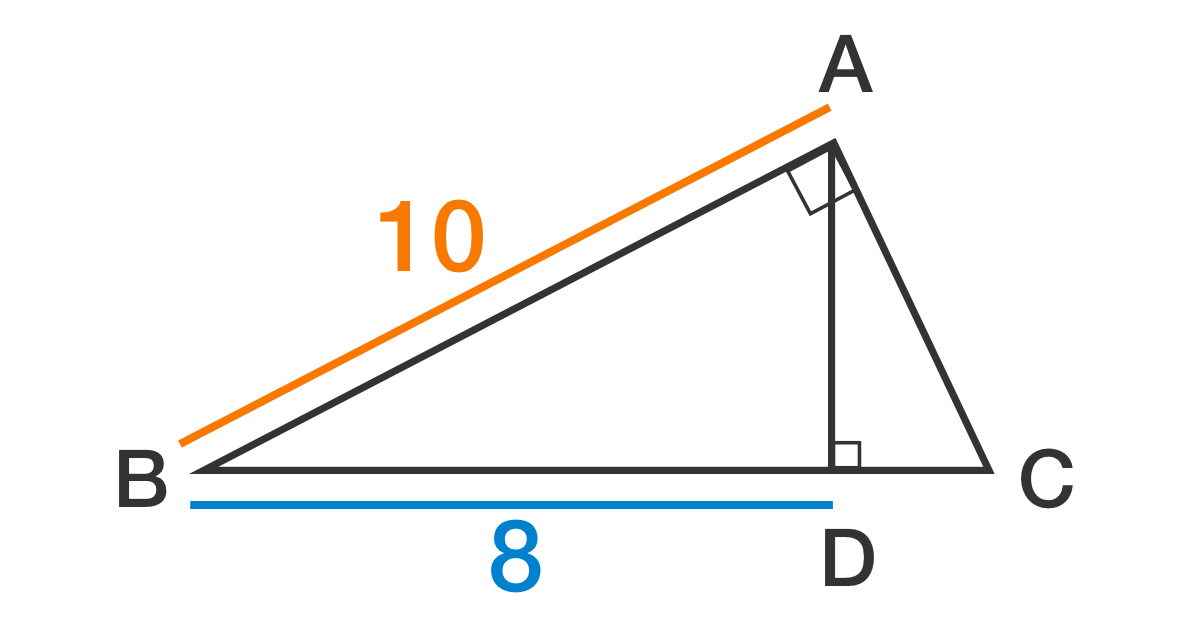# Pythagoras is Always Right

Geometry Level 2

$\triangle ABC$ is a right triangle and line segment $AD$ is an altitude.
If $AB = 10$ and $BD = 8$, then what is the area of $\triangle ABC$?×

Problem Loading...

Note Loading...

Set Loading...